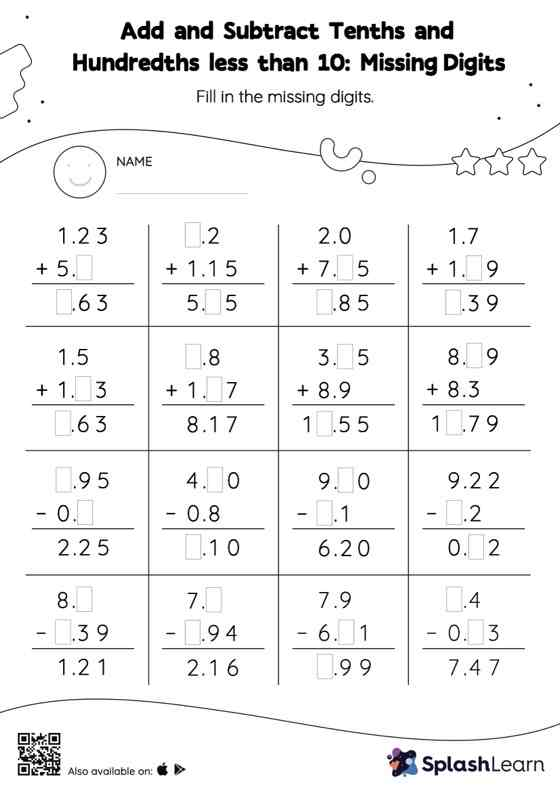# Add and Subtract Tenths and Hundredths less than 10: Missing Digits Worksheet

Home > Add and Subtract Tenths and Hundredths less than 10: Missing DigitsThis worksheet challenges young mathematicians to hone their math skills by solving a set of problems on adding and subtracting tenths and hundredths less than 10. In this worksheet, students add and subtract decimals by aligning the decimal points and using zero as a placeholder. They then use the relationship between addition and subtraction to find the missing number in the add and subtract tenths and hundredths less than 10 worksheet. In each problem, the numbers are laid out in the vertical format. In this format, pair of digits in each successive place are tackled one by one. This helps students follow a structured approach.